• 针对自由项为几类常见类型的三阶常系数非齐次线性微分方程，得到了求此类微分方程的特解公式，使求三阶常系数非齐次线性微分方程特解更加简易。
• ·基础数学· 基于 Matlab 常系数线性微分方程组的求解* 严水仙 (赣南师范大学 数学与计算机科学学院，江西 赣州 341000) 摘 要: 在微分方程课程教学中，常系数线性微分方程组可以通过线性代数的理论、矩阵指数、...
·基础数学· 基于 Matlab 常系数线性微分方程组的求解* 严水仙 (赣南师范大学 数学与计算机科学学院，江西 赣州 341000) 摘 要: 在常微分方程课程教学中，常系数线性微分方程组可以通过线性代数的理论、矩阵指数、拉普拉斯变 换等方法进行求解． 本文主要叙述利用 Matlab 数学软件在求解常系数线性微分方程组中的应用．关键词: 常系数线性微分方程;Matlab;矩阵指数 中图分类号: O175 文献标志码: A 文章编号:1004 －8332(2018)03 －0010 －05 微分方程课程是高校不少理工科专业(如数学、力学、控制等) 的重要基础理论课程． 常微分方程是描述自然科学、工程技术和社会科学中的运动、演化和变化规律的重要连续型模型． 物理、化学、材料、医学、经济学等领域中的许多原理和规律都可以描述成相应的微分方程，如生物种群中的生态平衡、流行病存在的阈值定理、化学反应中的稳定性、遗传基因变异、股票的涨幅趋势、利率的浮动、市场均衡价格的变化等． 描述、认识和分析其中的规律可以通过研究相应的微分方程数学模型来实现．［1］ 在微分方程的理论中，线性微分方程组是非常值得重视的一部分内容，它是了解并掌握非线性微分方程、非线性动力系统、非线性控制等课程的基础． 常系数线性微分方程组的求解是线性微分方程组理论中最简单、最直观的部分，熟悉并掌握常系数线性微分方程的求解将有利于更好的理解线性系统的基本理论． Matlab 是由美国的 Cleve Moler 博士等［2 －3］于 1980 年提出的以矩阵运算为基础，把计算、程序设计等融合到了一个简单易用的交互式工作环境中． 可实现工程计算、算法研究、符号运算、建模和仿真、原型开发、数据分析及可视化、科学和工程绘图、应用程序设计等功能． Matlab 强大的运算功能和图形使其成为目前世界上应用最为广泛的科学计算软件之一，在教学中能快速的计算方程的解并描绘直观的几何图形．［4 －6］鉴于此，本文主要介绍借助于 Matlab 来求解常系数线性微分方程组，通过利用 Matlab 命令，计算系数矩阵的特征值、特征向量、矩阵指数求解线性微分方程组． 1 常系数线性微分方程的基本理论［1］ 定理 1［1］ 如果 A(t) 是 n × n 阶矩阵函数， f(t) 是 n 维列向量函数． 它们都在区间 a  t  b 上连续，则 对区间 a  t  b 上的任意 t0 ∈［ a， b］及任一常数 n 维列向量 η ，方程组 x' = A(t)x + f(t) (1) 存在唯一解 φ(t)，定义于整个区间 a  t  b 上，且满足初值条件 φ(t0) = η．定理 2［1］ 齐次线性微分方程组 x' = A(t)x 一定存在 n 个线性无关的解 x1(t)， x2(t)，…， xn(t)． 定理 3［1］ 齐次线性微分方程组 x' = A(t)x 一定存在一个基解矩阵 Φ(t)． 如果 ψ(t) 是方程组的任意解，那么 ψ(t) = Φ(t)c， (2) 这里 c 是确定的 n 维常数列向量． 2018 年 赣南师范大学学报 №． 3 第三期 Journal of Gannan Normal University May． 2018 * 收稿日期:2017 －12 －12 DOI:10． 13698/j． cnki． cn36 －1346/c． 2018． 03． 003 基金项目: 江西省教育厅科学技术研究项目(GJJ170816)． 作者简介: 严水仙(1981 － )，男，江西省高安市人，赣
展开全文• 给出了常系数线性微分方程组...以广义特征向量链、指数矩阵和矩阵的秩为工具,分3种情形讨论了重根情形下常系数线性微分方程组的矩阵表示,建立了统一的代数结构,并对后2种情形,给出了相应的实例,以说明方法的有效性。
• 1. 引言 2. 准备知识 3. 系数齐次线性微分方程和欧拉方程 3.1 系数齐次线性微分方程的 3.2 Euler方程 4. 非齐次线性微分方程(比较系数法) ...在文献的4.2节，详细介绍了常系数线性微分方程的解法，对...


1. 引言
2. 准备知识
3. 常系数齐次线性微分方程和欧拉方程
3.1 常系数齐次线性微分方程的解
3.2 Euler方程

4. 非齐次线性微分方程(比较系数法)
4.1 形式 I
4.2 形式 II
4.3 Euler方程的另一种解法

参考文献

1. 引言

本文主要讲常系数线性微分方程的特征值法做了总结。在文献的4.2节，详细介绍了常系数线性微分方程的解法，对特征方程根的各种情况（实根或复根&根的重数）进行分类讲解，但由于分类过于仔细，使得读者对根的情况的记忆比较困难，本文致力于将特征根的各种情形统一处理，便于对微分方程解进行记忆.

2. 准备知识

本节所有的研究都是围绕着方程
dnxdtn+a1(t)dn−1xdtn−1+⋯+an−1(t)dxdt+an(t)x=f(x)(1)$\frac{{d}^{n}x}{d{t}^{n}}+{a}_{1}\left(t\right)\frac{{d}^{n-1}x}{d{t}^{n-1}}+\cdots +{a}_{n-1}\left(t\right)\frac{dx}{dt}+{a}_{n}\left(t\right)x=f\left(x\right)\phantom{\rule{2em}{0ex}}\left(1\right)$
\frac{d^nx}{d t^n}+a_1(t)\frac{d ^{n-1}x}{d t^{n-1}}+ \cdots +a_{n-1}(t)\frac{d x}{d t}+a_n(t)x=f(x)

进行的．其中 ai(t)(i=1,2,⋯,n)${a}_{i}\left(t\right)\left(i=1,2,\cdots ,n\right)$$a_i(t)(i=1,2,\cdots,n)$ 及 f(t)$f\left(t\right)$$f(t)$ 都是区间 [a,b]$\left[a,b\right]$$[a, b]$ 上的连续函数.
如果{} f(t)≡0$f\left(t\right)\equiv 0$$f(t)\equiv 0$，则方程(1)变为
dnxdtn+a1(t)dn−1xdtn−1+⋯+an−1(t)dxdt+an(t)x=0(2)(5)$\begin{array}{}\text{(5)}& \frac{{d}^{n}x}{d{t}^{n}}+{a}_{1}\left(t\right)\frac{{d}^{n-1}x}{d{t}^{n-1}}+\cdots +{a}_{n-1}\left(t\right)\frac{dx}{dt}+{a}_{n}\left(t\right)x=0\phantom{\rule{2em}{0ex}}\left(2\right)\end{array}$\begin{equation}
\frac{d^nx}{d t^n}+a_1(t)\frac{d^{n-1}x}{dt^{n-1}}+ \cdots +a_{n-1}(t)\frac{d x}{d t}+a_n(t)x=0 \qquad (2)
\end{equation}
设 K=α+$K=\alpha +$$K=\alpha +$iβ$\beta$$\beta$ 是任意复数，这里 α,β$\alpha ,\beta$$\alpha,\beta$ 是实数，t$t$$t$ 为实变量，那么有
eKt=e(α+iβ)t=eαt(cosβt+isinβt)(3)(6)$\begin{array}{}\text{(6)}& {e}^{Kt}={e}^{\left(\alpha +\text{i}\beta \right)t}={e}^{\alpha t}\left(\mathrm{cos}\beta t+\text{i}\mathrm{sin}\beta t\right)\phantom{\rule{2em}{0ex}}\left(3\right)\end{array}$\begin{equation}\label{dingyi}
e^{Kt} = e^{(\alpha + \text{i}\beta )t}=e^{\alpha t}(\cos{\beta t} + \text{i}\sin{\beta t})\qquad (3)
\end{equation}
此公式可通过泰勒展开进行验证．
定理1.1 如果方程(2)中所有系数 ai(t)(i=1,2,⋯,n)${a}_{i}\left(t\right)\left(i=1,2,\cdots ,n\right)$$a_i(t)(i=1,2,\cdots,n)$ 都是实值函数，而 x=z(t)=φ(t)+iψ(t)$x=z\left(t\right)=\phi \left(t\right)+\text{i}\psi \left(t\right)$$x=z(t)=\varphi(t)+\text{i}\psi(t)$ 是方程的复值解，则z(t)$z\left(t\right)$$z(t)$ 的实部 φ(t)$\phi \left(t\right)$$\varphi(t)$,虚部 ψ(t)$\psi \left(t\right)$$\psi(t)$ 和共轭复数z¯¯¯(t)$\overline{z}\left(t\right)$$\overline{z}(t)$ 也都是方程(2)的解．
定理1.2若方程
dnxdtn+a1(t)dn−1xdtn−1+⋯+an−1(t)dxdt+an(t)x=u(t)+iv(t)$\frac{{d}^{n}x}{d{t}^{n}}+{a}_{1}\left(t\right)\frac{{d}^{n-1}x}{d{t}^{n-1}}+\cdots +{a}_{n-1}\left(t\right)\frac{dx}{dt}+{a}_{n}\left(t\right)x=u\left(t\right)+iv\left(t\right)$
\frac{d ^nx}{d t^n}+a_1(t)\frac{d ^{n-1}x}{d t^{n-1}}+ \cdots +a_{n-1}(t)\frac{d x}{d t}+a_n(t)x=u(t)+iv(t)

有复值解x=U(t)+iV(t)$x=U\left(t\right)+\text{i}V\left(t\right)$$x=U(t)+\text{i}V(t)$，这里ai(t)(i=1,2,⋯,n)${a}_{i}\left(t\right)\left(i=1,2,\cdots ,n\right)$$a_i(t)(i=1,2,\cdots,n)$ 及U(t),V(t)$U\left(t\right),V\left(t\right)$$U(t),V(t)$ 都是实函数，那么这个解的实部U(t)$U\left(t\right)$$U(t)$ 和虚部V(t)$V\left(t\right)$$V(t)$ 分别是方程
dnxdtn+a1(t)dn−1xdtn−1+⋯+an−1(t)dxdt+an(t)x=u(t)$\frac{{d}^{n}x}{d{t}^{n}}+{a}_{1}\left(t\right)\frac{{d}^{n-1}x}{d{t}^{n-1}}+\cdots +{a}_{n-1}\left(t\right)\frac{dx}{dt}+{a}_{n}\left(t\right)x=u\left(t\right)$
\frac{d ^nx}{d t^n}+a_1(t)\frac{d ^{n-1}x}{d t^{n-1}}+ \cdots +a_{n-1}(t)\frac{d x}{d t}+a_n(t)x=u(t)

和
dnxdtn+a1(t)dn−1xdtn−1+⋯+an−1(t)dxdt+an(t)x=v(t)$\frac{{d}^{n}x}{d{t}^{n}}+{a}_{1}\left(t\right)\frac{{d}^{n-1}x}{d{t}^{n-1}}+\cdots +{a}_{n-1}\left(t\right)\frac{dx}{dt}+{a}_{n}\left(t\right)x=v\left(t\right)$
\frac{d ^nx}{d t^n}+a_1(t)\frac{d ^{n-1}x}{d t^{n-1}}+ \cdots +a_{n-1}(t)\frac{d x}{d t}+a_n(t)x=v(t)

的解．
注：上面两个定理保证了下述内容的正确性．定理1.1和定理1.2均来自文献.

3. 常系数齐次线性微分方程和欧拉方程

3.1 常系数齐次线性微分方程的解

设齐次线性微分方程中所有系数都是常数，即方程有如下形状
L[x]≡dnxdtn+a1dn−1xdtn−1+⋯+an−1dxdt+anx=0(4)(7)$\begin{array}{}\text{(7)}& L\left[x\right]\equiv \frac{{d}^{n}x}{d{t}^{n}}+{a}_{1}\frac{{d}^{n-1}x}{d{t}^{n-1}}+\cdots +{a}_{n-1}\frac{dx}{dt}+{a}_{n}x=0\phantom{\rule{2em}{0ex}}\left(4\right)\end{array}$\begin{equation}\label{fangcheng3}
L[x]\equiv \frac{d ^nx}{d t^n}+a_1\frac{d ^{n-1}x}{d t^{n-1}}+ \cdots +a_{n-1}\frac{d x}{d t}+a_nx=0 \qquad (4)
\end{equation}
其中 a1,a2,⋯,an${a}_{1},{a}_{2},\cdots ,{a}_{n}$$a_1,a_2,\cdots,a_n$ 为常数．
按照前面的理论，为了求方程(4)的通解，只需求其基本解组．回顾一阶常系数齐次微分方程
dxdt+ax=0$\frac{dx}{dt}+ax=0$
\frac{d x}{d t}+ax=0

已知，它有形如 x=e−at$x={e}^{-at}$$x=e^{-at}$ 的解，且其通解就是 x=ce−at$x=c{e}^{-at}$$x=ce^{-at}$．这就启发我们对方程(3)也去试求指数函数形式的解
x=eλt(5)(8)$\begin{array}{}\text{(8)}& x={e}^{\lambda t}\phantom{\rule{2em}{0ex}}\left(5\right)\end{array}$\begin{equation}\label{fangcheng4}
\end{equation}
其中 λ$\lambda$$\lambda$ 是待定常数，可以是实数，也可以是复数．
注意到
L[eλt]=dneλtdtn+a1dn−1eλtdtn−1+⋯+an−1deλtdt+aneλt=(λn+a1λn−1+⋯+an−1+an)eλt≡F(λ)eλt$\begin{array}{rl}L\left[{e}^{\lambda t}\right]& =\frac{{d}^{n}e\lambda t}{d{t}^{n}}+{a}_{1}\frac{{d}^{n-1}e\lambda t}{d{t}^{n-1}}+\cdots +{a}_{n-1}\frac{de\lambda t}{dt}+{a}_{n}e\lambda t\\ & =\left({\lambda }^{n}+{a}_{1}{\lambda }^{n-1}+\cdots +{a}_{n-1}+{a}_{n}\right){e}^{\lambda t}\equiv F\left(\lambda \right){e}^{\lambda t}\end{array}$\begin{align*}
L[e^{\lambda t}] & =\frac{d ^n e{\lambda t}}{d t^n}+a_1\frac{d ^{n-1} e{\lambda t}}{d t^{n-1}}+ \cdots +a_{n-1}\frac{d e{\lambda t}}{d t}+a_n e{\lambda t} \\
&=(\lambda ^n +a_1\lambda ^{n-1} + \cdots + a_{n-1} + a_n)e^{\lambda t}\equiv F(\lambda)e^{\lambda t}
\end{align*}
其中F(λ)=λn+a1λn−1+⋯+an−1+an$F\left(\lambda \right)={\lambda }^{n}+{a}_{1}{\lambda }^{n-1}+\cdots +{a}_{n-1}+{a}_{n}$$F(\lambda)=\lambda ^n +a_1\lambda ^{n-1} + \cdots + a_{n-1} + a_n$，是 λ$\lambda$$\lambda$ 的 n$n$$n$ 次多项式．式(5)为方程(4)的解的充要条件是 λ$\lambda$$\lambda$ 是代数方程
F(λ)=λn+a1λn−1+⋯+an−1+an=0(6)(9)$\begin{array}{}\text{(9)}& F\left(\lambda \right)={\lambda }^{n}+{a}_{1}{\lambda }^{n-1}+\cdots +{a}_{n-1}+{a}_{n}=0\phantom{\rule{2em}{0ex}}\left(6\right)\end{array}$\begin{equation}\label{fangcheng5}
F(\lambda)=\lambda ^n +a_1\lambda ^{n-1} + \cdots + a_{n-1} + a_n=0\qquad (6)
\end{equation}
的根．称(6)为方程(4)的特征方程，它的根就称为特征根．
设方程(4)的某一特征根为λ(k$\lambda \left(k$$\lambda(k$重,k⩾1)$k⩾1\right)$$k\geqslant 1)$，则k$k$$k$ 重特征根λ$\lambda$$\lambda$ 对应于方程(4)的k$k$$k$ 个线性无关解为
eλt,teλt,t2eλt,⋯,tkeλt.${e}^{\lambda t},t{e}^{\lambda t},{t}^{2}{e}^{\lambda t},\cdots ,{t}^{k}{e}^{\lambda t}.$\begin{equation*}
e^{\lambda t},te^{\lambda t},t^2e^{\lambda t},\cdots,t^ke^{\lambda t}.
\end{equation*}
当λ$\lambda$$\lambda$ 为复数时，只需用欧拉公式(3)转化，可得到2k$2k$$2k$ 个解，而 λ$\lambda$$\lambda$ 的共轭λ¯¯¯$\overline{\lambda }$$\overline{\lambda}$ 用此办法转化时，也得到相同的2k$2k$$2k$ 个解，这与λ$\lambda$$\lambda$ 和λ¯¯¯$\overline{\lambda }$$\overline{\lambda}$ 对应2k$2k$$2k$ 个解的事实相符．

3.2 Euler方程

形如
xndnydxn+a1xn−1dn−1ydxn−1+⋯+an−1xdydx+any=0(7)(10)$\begin{array}{}\text{(10)}& {x}^{n}\frac{{d}^{n}y}{d{x}^{n}}+{a}_{1}{x}^{n-1}\frac{{d}^{n-1}y}{d{x}^{n-1}}+\cdots +{a}_{n-1}x\frac{dy}{dx}+{a}_{n}y=0\phantom{\rule{2em}{0ex}}\left(7\right)\end{array}$\begin{equation}\label{Euler=function}
x^n\frac{d ^ny}{d x^n} + a_1x^{n-1}\frac{d ^{n-1}y}{d x^{n-1}}+ \cdots +a_{n-1}x\frac{d y}{d x}+a_ny=0\qquad (7)
\end{equation}
的方程称为欧拉方程，这里a1,a2,⋯,an${a}_{1},{a}_{2},\cdots ,{a}_{n}$$a_1,a_2,\cdots,a_n$ 为常数．以y=xk$y={x}^{k}$$y=x^k$ 代入(7)，并约去因子xk${x}^{k}$$x^k$，就得到用来确定k$k$$k$ 的代数方程
k(k−1)⋯(k−n+1)+a1k(k−1)⋯(k−n+2)+⋯+an=0(8)(11)$\begin{array}{}\text{(11)}& k\left(k-1\right)\cdots \left(k-n+1\right)+{a}_{1}k\left(k-1\right)\cdots \left(k-n+2\right)+\cdots +{a}_{n}=0\phantom{\rule{2em}{0ex}}\left(8\right)\end{array}$\begin{equation}\label{fangcheng6}
\end{equation}
因此，方程(8)的m$m$$m$ 重根k0${k}_{0}$$k_0$ 对应于方程(7)的m$m$$m$ 个解为
xk0,xk0ln|x|,xk0ln2|x|,⋯,xk0lnm−1|x|.${x}^{{k}_{0}},{x}^{{k}_{0}}\mathrm{ln}|x|,{x}^{{k}_{0}}{\mathrm{ln}}^{2}|x|,\cdots ,{x}^{{k}_{0}}{\mathrm{ln}}^{m-1}|x|.$\begin{equation*}
x^{k_0}, x^{k_0}\ln|x|, x^{k_0}\ln^2|x|, \cdots, x^{k_0}\ln^{m-1}|x|.
\end{equation*}
当为复数时，只需使用欧拉公式转换即可．

4. 非齐次线性微分方程(比较系数法)

下面讨论常系数非齐次线性微分方程
L[x]≡dnxdtn+a1dn−1xdtn−1+⋯+an−1dxdt+anx=f(t)(9)(12)$\begin{array}{}\text{(12)}& L\left[x\right]\equiv \frac{{d}^{n}x}{d{t}^{n}}+{a}_{1}\frac{{d}^{n-1}x}{d{t}^{n-1}}+\cdots +{a}_{n-1}\frac{dx}{dt}+{a}_{n}x=f\left(t\right)\phantom{\rule{2em}{0ex}}\left(9\right)\end{array}$\begin{equation}\label{fangcheng7}
L[x]\equiv \frac{d ^nx}{d t^n}+a_1\frac{d ^{n-1}x}{d t^{n-1}}+ \cdots +a_{n-1}\frac{d x}{d t}+a_nx=f(t)\qquad(9)
\end{equation}
的解．这里a1,a2,⋯,an${a}_{1},{a}_{2},\cdots ,{a}_{n}$$a_1,a_2,\cdots,a_n$ 是常数，f(t)$f\left(t\right)$$f(t)$ 是连续函数．

4.1 形式 I

设f(t)=(b0tm+b1tm−1+⋯+bm−1t+bm)eλt$f\left(t\right)=\left({b}_{0}{t}^{m}+{b}_{1}{t}^{m-1}+\cdots +{b}_{m-1}t+{b}_{m}\right){e}^{\lambda t}$$f(t) = (b_0t^m + b_1t^{m-1} +\cdots+ b_{m-1}t + b_m)e^{\lambda t}$，其中λ$\lambda$$\lambda$ 及bi(i=1,2,⋯,n)${b}_{i}\left(i=1,2,\cdots ,n\right)$$b_i(i=1,2,\cdots,n)$ 为实常数．则方程(9)有形如
x~=tk(B0tm+B1tm−1+⋯+Bm−1t+Bm)eλt(10)(13)$\begin{array}{}\text{(13)}& \stackrel{~}{x}={t}^{k}\left({B}_{0}{t}^{m}+{B}_{1}{t}^{m-1}+\cdots +{B}_{m-1}t+{B}_{m}\right){e}^{\lambda t}\phantom{\rule{2em}{0ex}}\left(10\right)\end{array}$\begin{equation}\label{fangcheng8}
\tilde{x} = t^k(B_0t^m + B_1t^{m-1} +\cdots+ B_{m-1}t + B_m)e^{\lambda t}\qquad(10)
\end{equation}
的特解．其中k$k$$k$ 为特征方程F(λ)=0$F\left(\lambda \right)=0$$F(\lambda)=0$ 的根λ$\lambda$$\lambda$ 的重数(λ$\lambda$$\lambda$ 不是特征根时认为是0$0$$0$ 重)．而B0,B1,⋯,Bm${B}_{0},{B}_{1},\cdots ,{B}_{m}$$B_0,B_1,\cdots,B_m$ 是待定常数，只需将x~$\stackrel{~}{x}$$\tilde{x}$ 代入原方程，比较对应项的系数即可计算出B0,B1,⋯,Bm${B}_{0},{B}_{1},\cdots ,{B}_{m}$$B_0,B_1,\cdots,B_m$ ，也即求出了方程(9)的特解．

4.2 形式 II

设f(t)=[A(t)cosβt+B(t)sinβt]eαt$f\left(t\right)=\left[A\left(t\right)\mathrm{cos}\beta t+B\left(t\right)\mathrm{sin}\beta t\right]{e}^{\alpha t}$$f(t) = [A(t)\cos\beta t + B(t)\sin\beta t]e^{\alpha t}$．其中α,β$\alpha ,\beta$$\alpha,\beta$ 为常数，而 A(t),B(t)$A\left(t\right),B\left(t\right)$$A(t),B(t)$ 是关于t$t$$t$ 的实系数多项式，A(t)$A\left(t\right)$$A(t)$ 与B(t)$B\left(t\right)$$B(t)$ 的次数为m$m$$m$ ．则方程(9)有形如
x~=tk[P(t)cosβt+Q(t)sinβt]eαt(11)(14)$\begin{array}{}\text{(14)}& \stackrel{~}{x}={t}^{k}\left[P\left(t\right)\mathrm{cos}\beta t+Q\left(t\right)\mathrm{sin}\beta t\right]{e}^{\alpha t}\phantom{\rule{2em}{0ex}}\left(11\right)\end{array}$\begin{equation}\label{fangcheng9}
\tilde{x} = t^k[P(t)\cos\beta t+Q(t)\sin\beta t]e^{\alpha t}\qquad(11)
\end{equation}
的特解．这里k$k$$k$ 是为特征方程F(λ)=0$F\left(\lambda \right)=0$$F(\lambda)=0$ 的根α+iβ$\alpha +\text{i}\beta$$\alpha+\text{i}\beta$ 的重数，而P(t),Q(t)$P\left(t\right),Q\left(t\right)$$P(t),Q(t)$ 均为待定的带实系数的次数不超过m$m$$m$ 的t$t$$t$ 的多形式，将(11)代回(9)，通过比较对应项的系数即可求出P(t),Q(t)$P\left(t\right),Q\left(t\right)$$P(t),Q(t)$，也即求出了方程(9)的特解．

4.3 Euler方程的另一种解法

可用变换x=et(即t=lnx)$x={e}^{t}\left(\text{即}t=\mathrm{ln}x\right)$$x=e^t(\text{即} t=\ln x)$ 将Euler方程(7)转化为前述的非齐次线性微分方程，即可求解．

参考文献

 王高雄等. 常微分方程(第三版)[M]. 北京: 高等教育出版社, 2006. 
展开全文Euler方程
• 利用积分公式和微分逆算子法，推导出一类二阶常系数非齐次线性微分方程特解公式，进而得出求此类微分方程特解的简便方法。
• 1、二阶常系数齐次线性微分方程 2、二阶常系数非齐次线性微分方程
1、二阶常系数齐次线性微分方程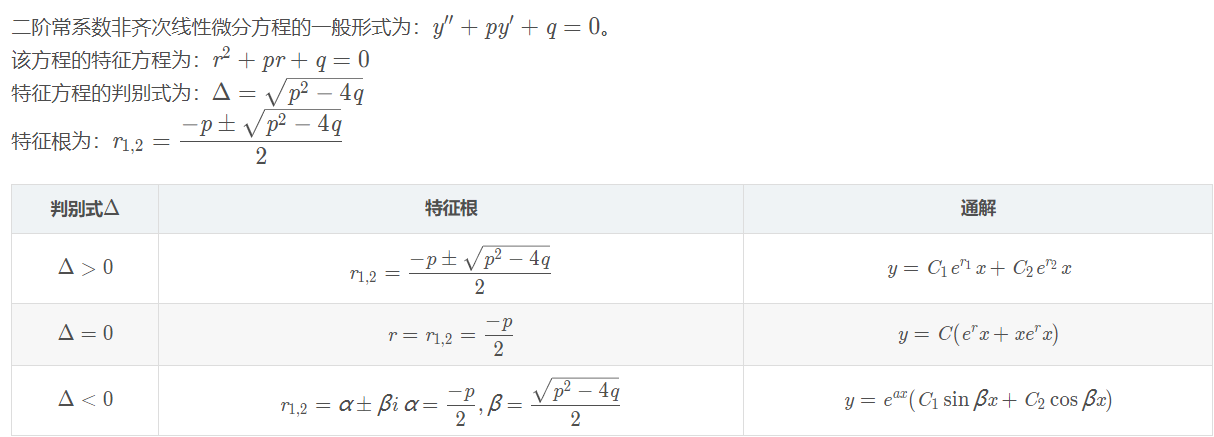2、二阶常系数非齐次线性微分方程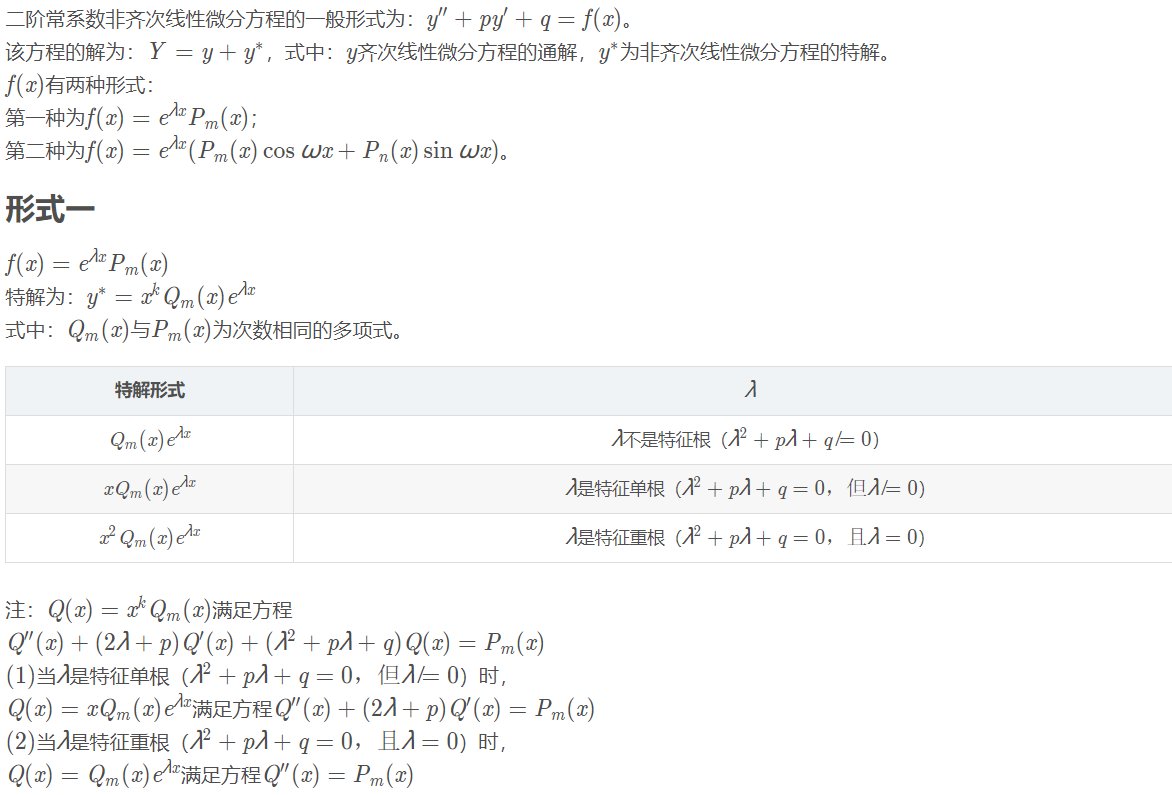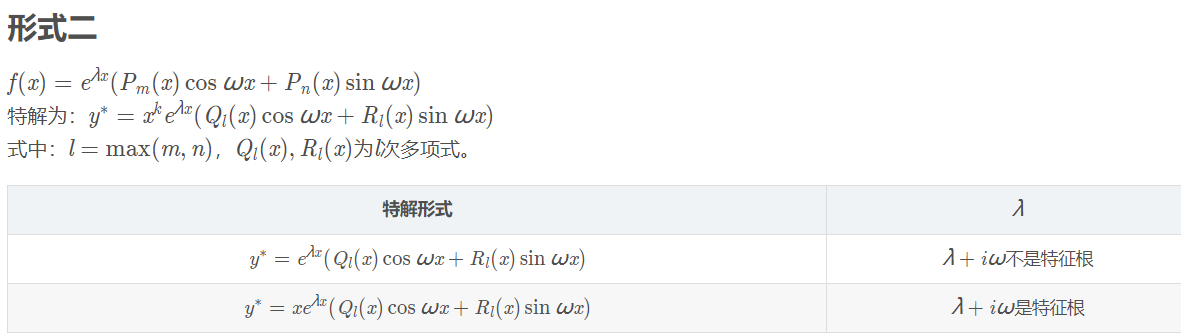展开全文• 利用上三角Toeplitz矩阵给出了常系数线性微分方程特解的表达式, 对于解常系数线性微分方程带来了很大方便.
• 基于Matlab常系数线性微分方程组的求解.pdf
• 前言 复值函数 常系数线性方程 法则 法则1 法则2 例题
前言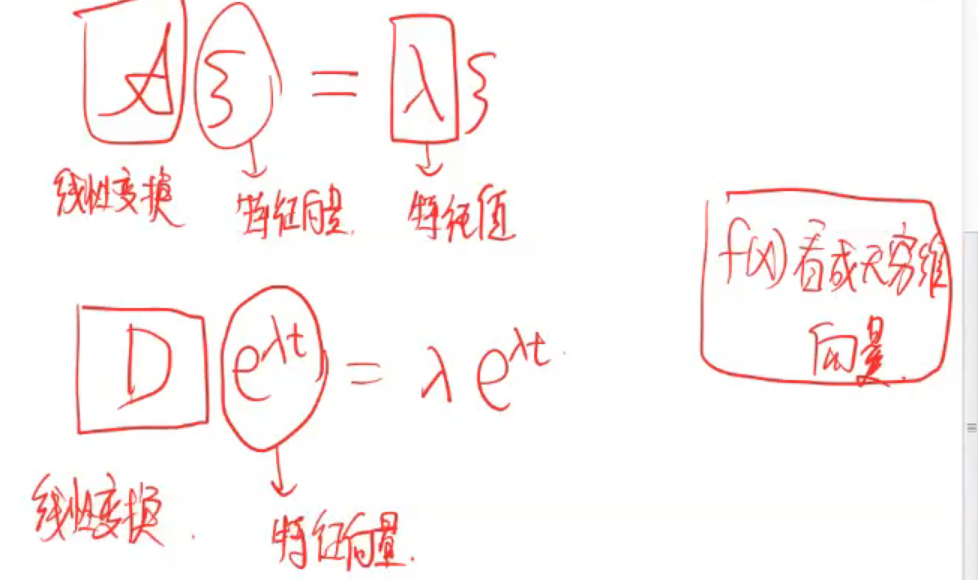复值函数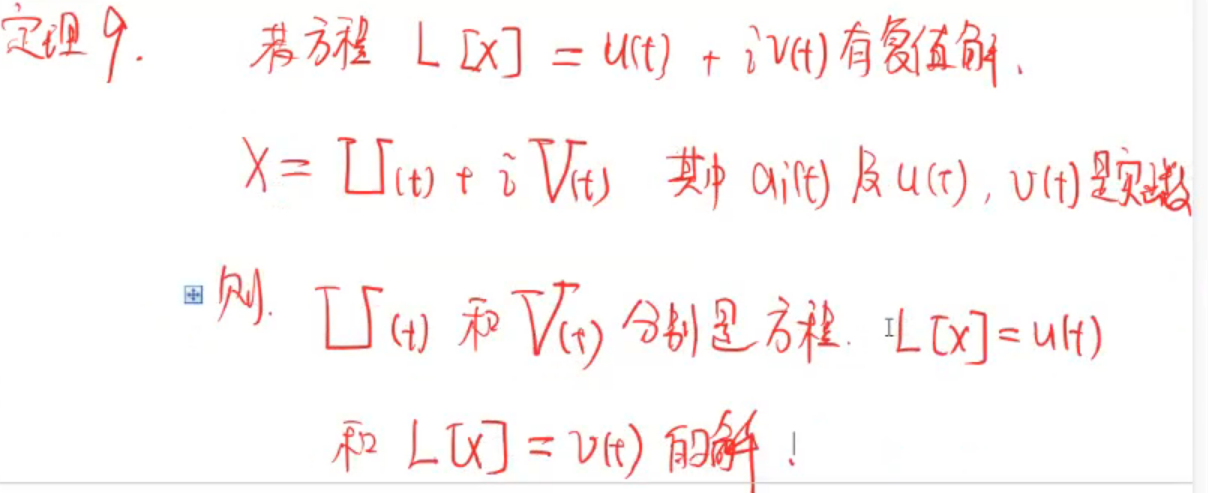常系数线性方程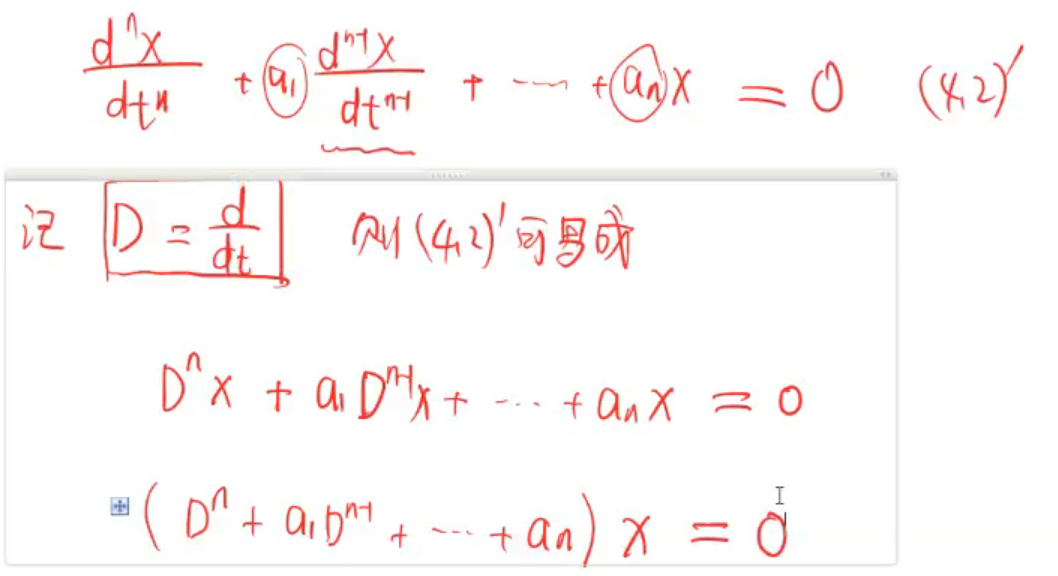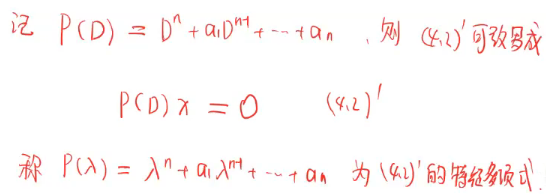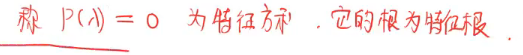法则
法则1法则2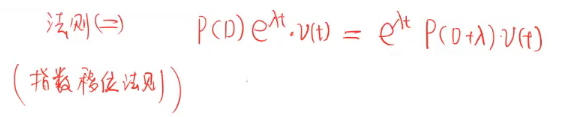例题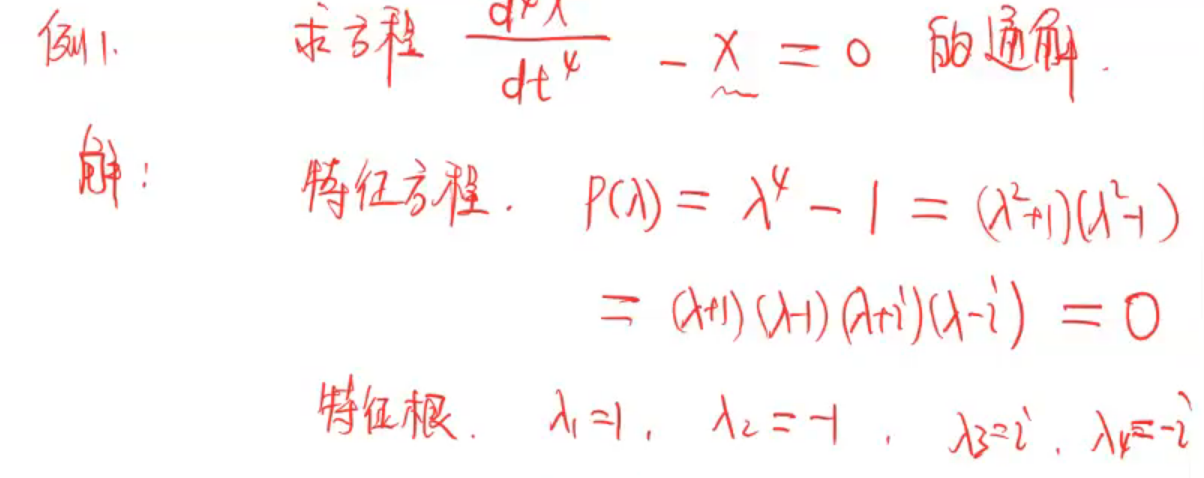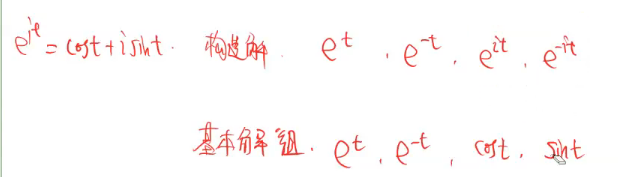展开全文• 本文介绍的常系数线性微分方程解题机是利用数学方法论的研究成果,摸拟人的数学思维过程,采用关系映射反演(RVII)方法,通过符号推理给出微分方程解析的数学软件文中介绍了研制该解题机的基本思想和实现技术,并给出了...
• 为了更简便地求出二阶常系数线性非齐次微分方程的一个特解，给出了一种直接积分方法．若已知二阶方程y″+py′+qy=f(x)的一个实特征根λ，可以使用直接积分的方法得到非齐次方程的一个特解y*=exp(-(λ+p)x)[∫(exp((2...
• 给出了常系数齐次线性微分方程组初值问题的一个求解公式,并由此推出常系数齐次线性差分方程组在给定的初始条件下的一个求解公式。
• *文中的微分方程均指代二阶常系数线性微分方程 二阶系数齐次线性微分方程的形式为： ay′′+by′+cy=0ay″+by′+cy=0ay'' + by' + cy = 0 由于是二阶线性微分方程，所以它有两个，记为y1、y2y1、y2y_1、y...高等数学
• 一、微分方程的基本概念 二、可分离变量的微分方程 三、齐次方程 1、齐次方程 2、可化为齐次的方程 ...七、常系数齐次线性微分方程 八、常系数非齐次线性微分方程 1、f(x)=eλxPm(x)型 2、f(x)=eλx[Plcosω人工智能 机器学习 数学
• 找到方程1的两个线性无关的，则它们的组合y=C1y1(x)+C2y2(x)是方程1的通 方案二 当r为常数时，y=erx 和它的n次导数只差一个常数因子，哦吼，想到了什么？似乎已经初步看到了希望的小火苗。 对y=erx 分别求1次，2...
• 微分方程的通解 = 对应的二阶常系数齐次线性微分方程通解 + 自身的一个特解 简单记为：通解 = 齐次通解 + 特解。 二阶常系数齐次线性微分方程通解的解法：二阶常系数齐次线性微分方程的通解 下面只需要解出微分方程...
• 给出了二阶常系数线性非齐次微分方程y+ay1+by=f（x）的多种解法，旨在介绍二阶方程的一些解题方法与解题思路。
• 二阶常系数线性微分方程是形如y''+py'+qy=f(x)的微分方程，其中p，q是实常数。自由项f(x)为定义在区间I上的连续函数，即y''+py'+qy=0时，称为二阶系数齐次线性微分方程。若函数y1和y2之比为常数，称y1和y2是线性...
• 利用引理，确定了n阶常系数非齐次线性微分方程特解的待定形式；简化了待定系数法的证明，使求特解更加简易。
• 利用方程组系数矩阵的特征根,给出二元常系数非齐次线性微分方程特解的一种求法。
• 研究了几类K阶整系数线性微分方程解的超级、零点收敛指数和零点超收敛指数,得到一些精确的结果.
• 例如：二阶线性微分方程 X"＋2Xˊ＋5X=0 ， X︱t=0 =0 ，Xˊ︱t=0 =0   这是个齐次二阶常系数微分方程，对应的初始条件为均0，意味着系统初始状态为0，由于系统无激励，系统的初始状态又为0，所以系统不运动，即X...
• 原文：https://www.q-math.com/?p=282...